/

### Enterprise Risk Management Formula Book Appendix A.2: Probability Distributions: Continuous (univariate) distributions (c) generalised Pareto, lognormal, Student’s t

Distribution name

Generalised Pareto distribution (GPD)

Common notation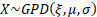Parameters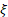= shape parameter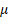= location parameter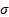= scale parameter (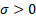)

Domain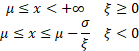Probability density function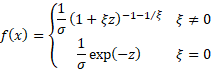where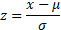Cumulative distribution function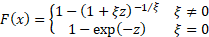Mean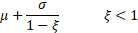Variance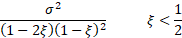Skewness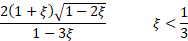(Excess) kurtosis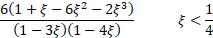GPD is used in the peaks over thresholds variant of extreme value theory

 Distribution name Lognormal distribution Common notation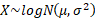Parameters= scale parameter ()= location parameter Domain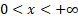Probability density function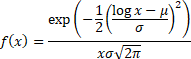Cumulative distribution function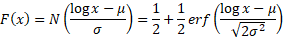Mean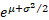Variance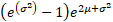Skewness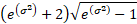(Excess) kurtosis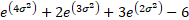Characteristic function No simple expression that is not divergent Other comments The median of a lognormal distribution is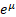and its mode is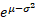.   The truncated moments of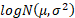are: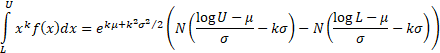Distribution name (Standard) Student’s t distribution Common notation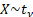Parameters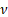= degrees of freedom (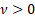, usuallyis an integer although in some situations a non-integralcan arise) Domain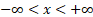Probability density function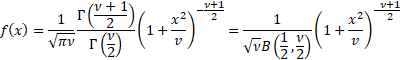Cumulative distribution function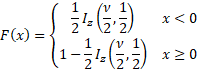where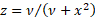Mean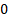Variance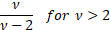Skewness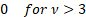(Excess) kurtosis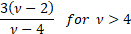Characteristic function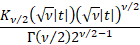where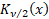is a Bessel function Other comments The Student’s t distribution (more simply the t distribution) arises when estimating the mean of a normally distributed population when sample sizes are small and the population standard deviation is unknown.   It is a special case of the generalised hyperbolic distribution.   Its non-central moments ifis even and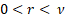are: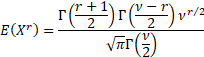Ifis even and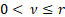then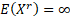, ifis odd andthen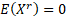and ifis odd andthen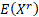is undefined.